# 为人脸添加特效的思路

1. 检测图像/视频中人脸的关键点
2. 调整特效图像的尺寸，让其匹配人脸
3. 确定人脸图像中的感兴趣区域（Region of Interest, ROI区域）
4. 消除特效图像中白色背景
5. 将特效图像置换到ROI区域上，实现特效添加效果
# 导入所需库
import cv2
import mediapipe as mp
import math
import matplotlib.pyplot as plt
import time


# 1. 获得人脸关键点

# 获取人脸关键点
def get_landmarks(image, face_mesh):
"""
:param image: ndarray图像
:param face_mesh: 人脸检测模型
:return:人脸关键点列表，如[{0:(x,y),1:{x,y},...},{0:(x,y),1:(x,y)}]
"""
landmarks = []
height, width = image.shape[0:2]
# 人脸关键点检测
results = face_mesh.process(cv2.cvtColor(image, cv2.COLOR_BGR2RGB))
# 解释检测结果
if results.multi_face_landmarks:
for face_landmarks in results.multi_face_landmarks:
i = 0
points = {}
# 根据图像的高度和宽度还原关键点位置
for landmark in face_landmarks.landmark:
x = math.floor(landmark.x * width)
y = math.floor(landmark.y * height)
points[i] = (x, y)
i += 1
landmarks.append(points)

return landmarks


math.floor()函数用来返回向下取整的数字,如下所示。

# math.floor()的作用
print(math.floor(0.8)) # 输出0
print(math.floor(4.7)) # 输出4


# 模型配置
mp_face_mesh = mp.solutions.face_mesh
face_mesh = mp_face_mesh.FaceMesh(static_image_mode=False,
max_num_faces=3,
refine_landmarks=True,
min_detection_confidence=0.5,
min_tracking_confidence=0.5)
# 读取图像
# 获取关键点
face_landmarks = get_landmarks(image, face_mesh)
# 输出第1个人脸，序号为3的关键点位置
print(face_landmarks)


# 2. 调整特效尺寸def process_effects(landmarks,icon_path, icon_name):
"""
:param landmarks: 检测到的人脸关键点列表
:param icon_path: 特效图像地址
:param icon_name: 特效名称
:return:处理好的特效图像、特效宽、特效高
"""
# 特效关键点，用于调整特效的尺寸
effect_landmarks = {"beard": ((landmarks, landmarks), (landmarks, landmarks)),
"eyeglass": ((landmarks, landmarks), (landmarks, landmarks)),

# 读取特效图像
# 选择特效关键点
pt1, pt2 = effect_landmarks[icon_name]
x, y, x_w, y_h = pt1, pt1, pt2, pt2
# 调整特效的尺寸
w, h = x_w - x, y_h - y
effect = cv2.resize(icon, (w, h))

return effect, w, h


# 3. ROI区域

• 选择该方法确定ROI区域，可以让特效随着中心点移动，在视频显示时效果更好。
• 同时需要注意的是ROI的尺寸与特效的尺寸要一致，才能进行替换，如果不一致，会出现报错。landmarks[p] - int(h / 2) + h - (landmarks[p] - int(h / 2))就是特效图像的高h；
landmarks[p] - int(w / 2) + w - (landmarks[p] - int(w / 2))就是特效图像的宽w。

p = 164 # 第164号关键点为中心
# ROI区域，w,h为特效的宽与高
roi = image[landmarks[p] - int(h / 2):landmarks[p] - int(h / 2) + h,
landmarks[p] - int(w / 2):landmarks[p] - int(w / 2) + w]


h = 373
print(int(h / 2)+int(h / 2))  # 该输出结果为372


image_copy = image.copy()
icon_name = "beard"
p = 164
for landmarks in face_landmarks:
# 调整特效尺寸
effect, w, h = process_effects(landmarks, "icons/"+icon_name+".png", icon_name)
# 以164号关键点为中心点，确定ROI区域
roi = image_copy[landmarks[p] - int(h / 2):landmarks[p] - int(h / 2) + h,
landmarks[p] - int(w / 2):landmarks[p] - int(w / 2) + w]
if roi.shape[0:2] == effect.shape[0:2]:
# 特效图像替换ROI区域
image_copy[landmarks[p] - int(h / 2):landmarks[p] - int(h / 2) + h,
landmarks[p] - int(w / 2):landmarks[p] - int(w / 2) + w] = effect

# 利用matplotlib展示
plt.figure(figsize=(10, 5))
plt.imshow(image_copy[:, :, ::-1])# 4. 消除特效图像中白色背景

## 第一种方法：像素值替换# 循环特效图像中每一个像素点，替换大于阈值的像素值
def swap_non_effcet1(effect,roi,threshold=240):
"""
:param effect: 特效图像
:param roi: ROI区域
:param threshold: 阈值
"""
for h in range(effect.shape):
for w in range(effect.shape):
for k in range(3):
if effect[h][w][k] > threshold:
effect[h][w][k] = roi[h][w][k]## 第二种方法：图像运算

### 二进制“与”运算

“与”运算的规则：1&1=1，1&0=0，0&1=0，0&0=0。

• 任何数据与0的二进制“与运算”都是0；
• 任何数据与1的二进制“与运算”都能保留原数据信息。

• 199的二进制是11000111
• 0的二进制是00000000
• 则 199 & 0 = 11000111 & 00000000 = 00000000。
• 255的二进制是11111111
• 则 199 & 255 = 11000111 & 11111111 = 11000111opencv中实现二进制与运算的方法为cv2.bitwise_and()

# 将特效图像(effect)与ROI做二进制“与运算”
effect = cv2.bitwise_and(effect, roi)### 处理彩色特效

image_copy = image.copy()
# 获取特效图像和ROI区域
p = 164
effect, w, h = process_effects(landmarks, "icons/beard_color.png", icon_name)
# 以164号关键点为中心点，确定ROI区域
roi = image_copy[landmarks[p] - int(h / 2):landmarks[p] - int(h / 2) + h,
landmarks[p] - int(w / 2):landmarks[p] - int(w / 2) + w]

plt.imshow(effect[:, :, ::-1])
print(effect.shape) # 获得图像尺寸
print(effect[200,200]) # (200,200)位置的像素值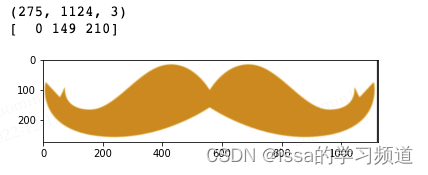cv2.cvtColor(img,cv2.COLOR_BGR2GRA)可以将彩色图像图像转换为灰度图像。

# 图像灰度化
effect2gray = cv2.cvtColor(effect, cv2.COLOR_BGR2GRAY)
plt.imshow(effect2gray,'gray')
print(effect2gray.shape) # 获得图像尺寸
print(effect2gray[200,200]) # (200,200)位置的像素值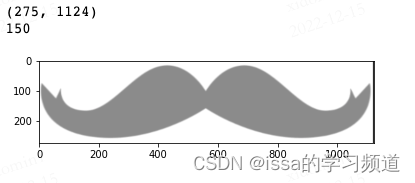cv2.threshold(img, thresh, maxval, type)参数说明：

• img:原始灰度图像
• thresh:阈值
• maxval:最大值，如255.
• type:阈值类型,定义了如何处理数据与阈值的关系，如当type = cv2.THRESH_BINARY时，原始图像中灰度值如果大于阈值，将该灰度值转换为最大值，否则转换为0
• 返回值:阈值和二值化后的图像。
# 图像二值化（阈值为180）
ret, effect2wb = cv2.threshold(effect2gray, 180, 255, cv2.THRESH_BINARY)
plt.imshow(effect2wb,'gray')
print(effect2wb.shape) # 获得图像尺寸
print(effect2wb[100,100]) # (100,100)位置的像素值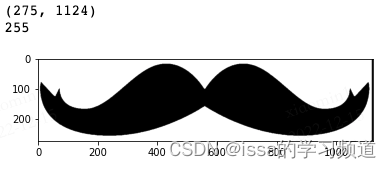dst(I)=src1(I) & src2(I) if mask(I)≠0 dst为输出图像。

effectwb = cv2.bitwise_and(roi,roi,mask = effect2wb)
plt.imshow(effectwb[:, :, ::-1])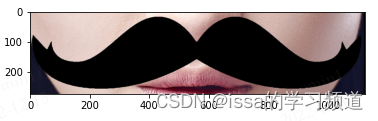opencv中提供了“叠加”的方法cv2.add(src1,src2),任何像素值与0相加都能获得像素值本身（如230 + 0 = 230）。叠加时，需要保证“相加”的两个图像有相同的尺寸和通道数。

a = cv2.add(effectwb,effect)
plt.imshow(a[:, :, ::-1])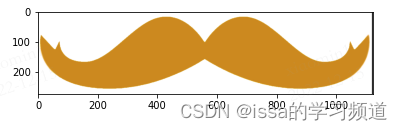# 首先反转二值化后的特效
effect2wb_ne = cv2.bitwise_not(effect2wb)
plt.imshow(effect2wb_ne,'gray')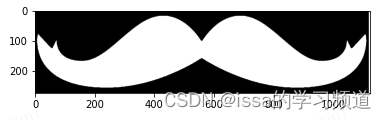# 然后与运算处理彩色特效
plt.imshow(effectcolor[:, :, ::-1])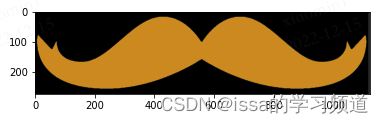# 最后将处理后的彩色特效与黑色特效进行叠加
plt.imshow(effect_final[:, :, ::-1])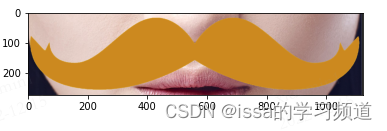def swap_non_effcet2(effect, roi, threshold=240):
"""
:param effect: 特效图像
:param roi: ROI区域
:param threshold: 阈值
:return: 消除背景后的特效图像
"""

# （1）特效图像灰度化
effect2gray = cv2.cvtColor(effect, cv2.COLOR_BGR2GRAY)
# （2）特效图像二值化
ret, effect2wb = cv2.threshold(effect2gray, threshold, 255, cv2.THRESH_BINARY)
# （3）消除特效的白色背景

# （4）反转二值化后的特效
effect2wb_ne = cv2.bitwise_not(effect2wb)
# （5）处理彩色特效
# (6) 组合彩色特效与黑色特效

return effect_final


# 添加特效完整代码

# 模型配置
mp_face_mesh = mp.solutions.face_mesh
face_mesh = mp_face_mesh.FaceMesh(static_image_mode=False,  # 静态图片设置为False,视频设置为True
max_num_faces=3,  # 能检测的最大人脸数
refine_landmarks=True,  # 是否需要对嘴唇、眼睛、瞳孔的关键点进行定位，
min_detection_confidence=0.5,  # 人脸检测的置信度
min_tracking_confidence=0.5)  # 人脸追踪的置信度（检测图像时可以忽略）

# 读取图像
# 获取关键点
face_landmarks = get_landmarks(image, face_mesh)

# ROI区域中心关键点
center = {"beard": 164, "eyeglass": 168, "halfmask": 9}

# 处理特效
icon_name = "beard"
for landmarks in face_landmarks:
effect, w, h = process_effects(landmarks, "icons/"+icon_name+".png", icon_name)
# 确定ROI
p = center[icon_name]
roi = image[landmarks[p] - int(h / 2):landmarks[p] - int(h / 2) + h,
landmarks[p] - int(w / 2):landmarks[p] - int(w / 2) + w]

if effect.shape[:2] == roi.shape[:2]:
# 消除特效图像中的白色背景区域
s = time.time()
# 第一种方法
# swap_non_effcet1(effect,roi,240)
# 第二种方法
effect = swap_non_effcet2(effect, roi,240)

print(time.time()-s) # 计算处理时间

# 将处理好的特效添加到人脸图像上
image[landmarks[p] - int(h / 2):landmarks[p] - int(h / 2) + h,
landmarks[p] - int(w / 2):landmarks[p] - int(w / 2) + w] = effect

# 利用matplotlib展示
plt.figure(figsize=(30, 10))
plt.imshow(image[:, :, ::-1])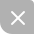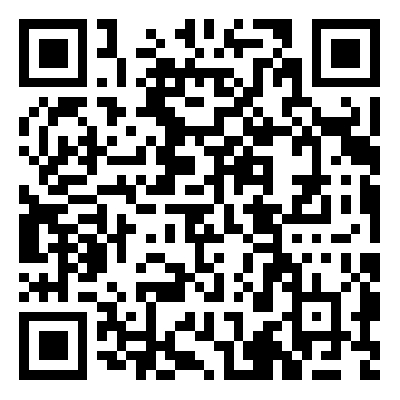UP更新不错过~
01-27391
08-212527
02-28492
12-14652
11-02623
12-214816
11-23633
06-302718
12-282222
07-27674
02-01127
12-241093
08-057316
09-09782
03-284959
11-02183

### “相关推荐”对你有帮助么？

•非常没帮助
•没帮助
•一般
•有帮助
•非常有帮助被折叠的  条评论 为什么被折叠?到【灌水乐园】发言issa的学习频道

¥2 ¥4 ¥6 ¥10 ¥20余额支付 (余额：-- )扫码支付获取中扫码支付点击重新获取扫码支付1.余额是钱包充值的虚拟货币，按照1:1的比例进行支付金额的抵扣。
2.余额无法直接购买下载，可以购买VIP、C币套餐、付费专栏及课程。余额充值